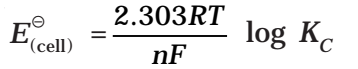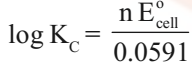## Nernst Equation

It relates electrode potential with the concentration of ions. Thus, the reduction potential increases with the increase in the concentration of ions. For a general electrochemical reaction of the type.Nernst equation can be given as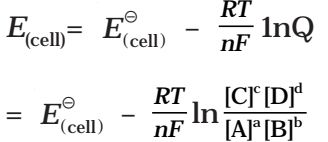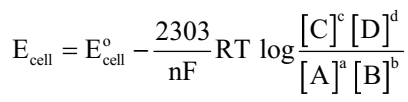Substituting the values of R and F we get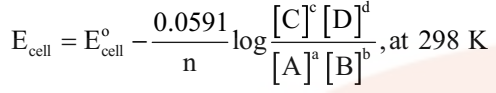## Application of Nernst Equation

### Equilibrium Constant from Nernst Equation:

For a Daniel cell, at equilibrium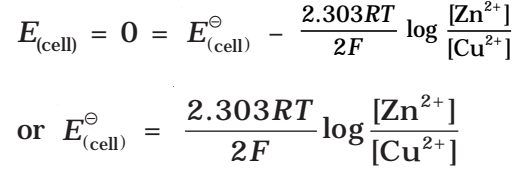But at equilibrium,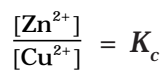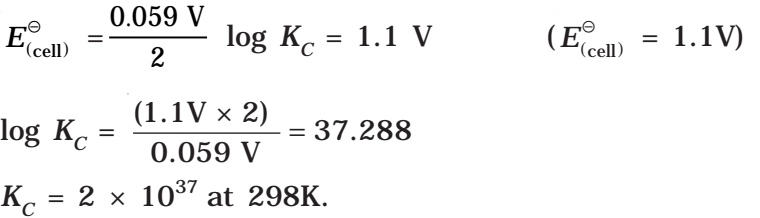In general,